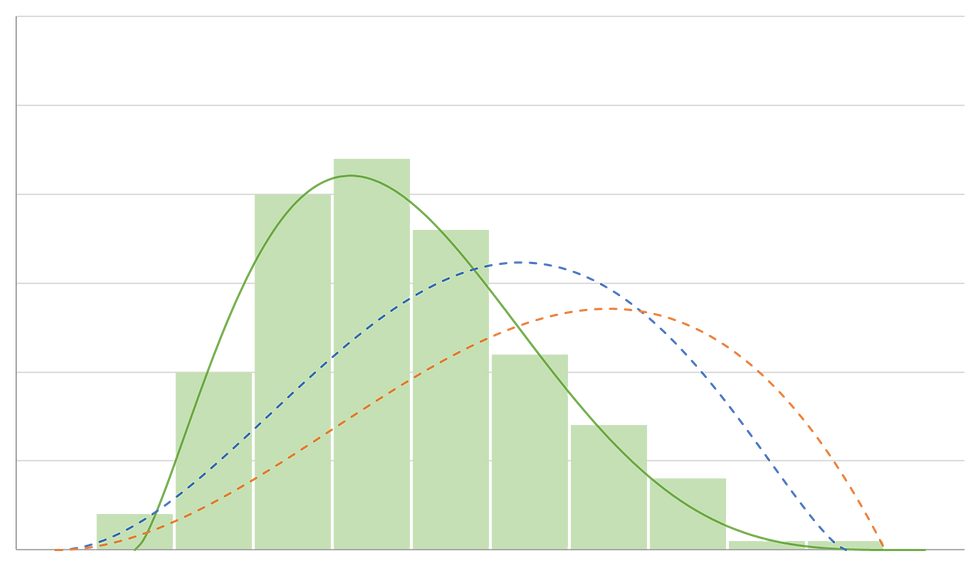top of page

# Using Monte Carlo Analysis in Technology Development: Quantifying risk due to uncertainty

“It is an inescapable fact that estimates of resource requirements for future systems are beset with uncertainty. The question is not whether uncertainty exists, but rather in determining the magnitude and nature of the uncertainty.” – Paul Dienemann, RAND Corporation

Uncertainty in decision-making

Cost models are built with layer upon layer of assumptions. We make assumptions about process parameters, equipment costs, and scaling factors. We make more assumptions about chemical prices, tax rates, and discount rates. We can usually estimate the uncertainty for each individual assumption intuitively, but understanding their combined effect is beyond us.

Understanding the magnitude and nature of uncertainty in our estimates is important in both project management and project evaluation. With quantitative information about uncertainty, we can:

• develop more accurate budgets, justifying contingency allowances with statistical metrics rather than rules of thumb;

• quantitatively evaluate and compare risk mitigation strategies;

• take organizational risk tolerance into consideration;

• make better decisions when choosing between project alternatives, especially those with similar ‘expected-case’ economics but differing degrees of uncertainty.

Consider the following example, in which two projects are compared based on net present value (NPV) (1). These projects could represent different processes, process configurations, or risk mitigation strategies. The uncertainty around each estimate is expressed as a probability distribution curve. The highest point on the curve represents the mode of the distribution, or the expected value. Note that if you were to only consider the expected values, you would be inclined to choose the better option in Case 1, but the worse option in Case 3. In Case 2, the options would appear interchangeable.Approaches to quantifying uncertainty

There are two general approaches to understanding cumulative uncertainty in a cost model: deterministic sensitivity analysis and probabilistic sensitivity analysis.

Deterministic sensitivity analysis brackets uncertainty with best and worst case scenarios. It is a valuable tool for comparing the impact of individual parameters, for example with a tornado diagram; but when all parameters are set to extreme cases, the results are often unrealistic. Deterministic sensitivity analysis gives us little information about the where the result is likely to fall within the range predicted.

Probabilistic sensitivity analysis, on the other hand, allows us to generate probability distributions like those in the diagram above, and thereby to quantitatively evaluate cumulative uncertainty and risk. The technique used for generating these probability distributions from a model is known as the Monte Carlo method, or Monte Carlo analysis.

The Monte Carlo method

A cost model has input parameters and output parameters. The Monte Carlo method is an iterative numerical algorithm that uses probability distributions for the input parameters to develop probability distributions for the output parameters. The process can be broken down into three phases as shown in the diagram below.In the first phase, we approximate a probability distribution functions (PDF ) for each input parameter. The PDFs can be derived from data (empirical or historical) or from expert estimates. The most common method for deriving PDFs from expert estimates is borrowed from the statistical project management tool PERT. In PERT, each input parameter is assigned a beta distribution based on three estimated values: minimum-case, maximum-case, and expected-case. The beta distribution is used because it has the qualitative characteristics we expect in a real, continuous variable; it is finite in range, unimodal, and has a bell-curve type shape, but it can also accommodate asymmetry. Reference 2 provides more detailed information about working with beta distributions in Excel, and sampling randomly from them.

In the second phase, we use random sampling from the PDFs to generate values for each input variable. We then plug these values into the model and save the results. This process is repeated until enough data points have been collected to define characterize the result distribution.

In the third phase, we aggregate and statistically analyze the result data. It can be represented graphically as a histogram, probability distribution, or cumulative probability distribution, or numerically using metrics like mean, mode, and confidence intervals.

Closing thoughts

To implement the Monte Carlo method, you will need an automated cost model, the right software tools, and the expertise to use them. However, the quality of the results will depend on the accuracy of your model and input probability distributions. In early-stage technology development, we are often relying on preliminary estimates and experiments, so uncertainty is bound to be high. For this reason, some organizations may be reluctant to engage in sensitivity analysis, or even to build a cost model in the first place.

There is some irony in this – to ignore uncertainty because it is too great. Uncertainty may be inescapable, but it is not necessarily unknowable. We need to make skillful decisions to succeed in technology development, balancing benefit and risk. We need to understand both sides of this equation if we are to choose winning projects, to develop robust management plans, and to pivot early to avoid dead-ends. Cost modeling with Monte Carlo analysis can help us do that.